# Information, amount of

An information-theoretical measure of the quantity of information contained in one random variable relative to another random variable. Letandbe random variables defined on a probability space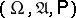and taking values in measurable spaces (cf. Measurable space)and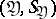, respectively. Let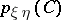,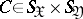, and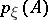,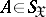,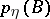,, be their joint and marginale probability distributions. If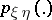is absolutely continuous with respect to the direct product of measures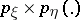, if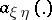is the (Radon–Nikodým) density ofwith respect to, and if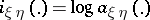is the information density (the logarithms are usually taken to base 2 or), then, by definition, the amount of information is given by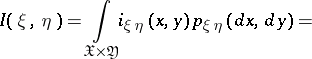Ifis not absolutely continuous with respect to, then, by definition.

In case the random variablesandtake only a finite number of values, the expression for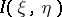takes the form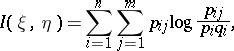whereare the probability functions of,and the pair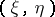, respectively. (In particular,is the entropy of.) In caseandare random vectors and the densities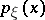,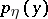andof,and the pair, respectively, exist, one hasIn general,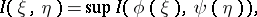where the supremum is over all measurable functions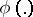and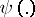with a finite number of values. The concept of the amount of information is mainly used in the theory of information transmission.

For references, see , ,

How to Cite This Entry:
Information, amount of. R.L. DobrushinV.V. Prelov (originator), Encyclopedia of Mathematics. URL: http://www.encyclopediaofmath.org/index.php?title=Information,_amount_of&oldid=12464
This text originally appeared in Encyclopedia of Mathematics - ISBN 1402006098